Next: Transformation of fields Up: Relativity and electromagnetism Previous: The electromagnetic field tensor

## The dual electromagnetic field tensor

We have seen that it is possible to write the components of the electric and magnetic fields as the components of a proper-4-tensor. Is it also possible to write the components of these fields as the components of some pseudo-4-tensor? It is obvious that we cannot identify the components of the proper-3-vector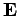with any of the components of a pseudo-tensor. However, we can represent the components ofin terms of those of an antisymmetric pseudo-3-tensorby writing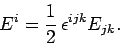(1491)

It is easily demonstrated that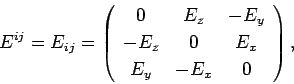(1492)

in a right-handed coordinate system.

Consider the dual electromagnetic field tensor,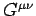, which is defined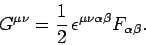(1493)

This tensor is clearly an antisymmetric pseudo-4-tensor. We have(1494)

plus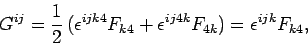(1495)

where use has been made of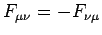. The above expression yields(1496)

It follows that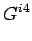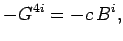(1497)(1498)

or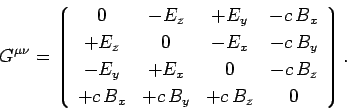(1499)

The above expression is, again, slightly misleading, since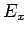stands for the component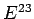of the pseudo-3-tensor, and not for an element of the proper-3-vector. Of course, in this case,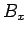really does represent the first element of the pseudo-3-vector. Note that the elements ofare obtained from those of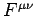by making the transformationand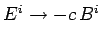.

The covariant elements of the dual electromagnetic field tensor are given by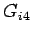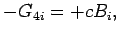(1500)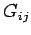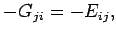(1501)

or(1502)

The elements of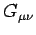are obtained from those of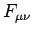by making the transformation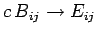and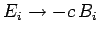.

Let us now consider the two Maxwell equations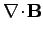(1503)(1504)

The first of these equations can be written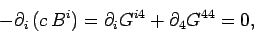(1505)

since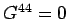. The second equation takes the form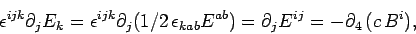(1506)

or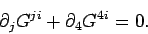(1507)

Equations (1505) and (1507) can be combined to give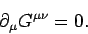(1508)

Thus, we conclude that Maxwell's equations for the electromagnetic fields are equivalent to the following pair of 4-tensor equations: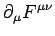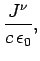(1509)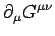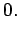(1510)

It is obvious from the form of these equations that the laws of electromagnetism are invariant under translations, rotations, special Lorentz transformations, parity inversions, or any combination of these transformations.Next: Transformation of fields Up: Relativity and electromagnetism Previous: The electromagnetic field tensor
Richard Fitzpatrick 2006-02-02